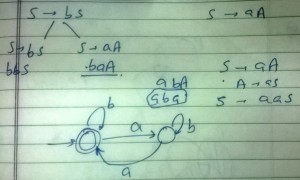# GATE | GATE IT 2006 | Question 29

Consider the regular grammar below
S → bS | aA | ϵ
A → aS | bA
The Myhill-Nerode equivalence classes for the language generated by the grammar are
(A) {w ∊ (a + b)* | #a(w) is even) and {w ∊ (a + b)* | #a(w) is odd}
(B) {w ∊ (a + b)* | #a(w) is even) and {w ∊ (a + b)* | #b(w) is odd}
(C) {w ∊ (a + b)* | #a(w) = #b(w) and {w ∊ (a + b)* | #a(w) ≠ #b(w)}
(D) {ϵ}, {wa | w ∊ (a + b)* and {wb | w ∊ (a + b)*}

Explanation: